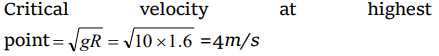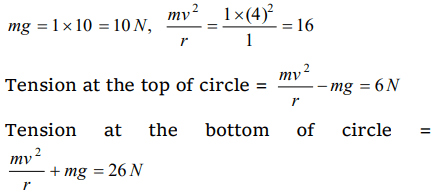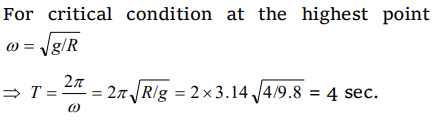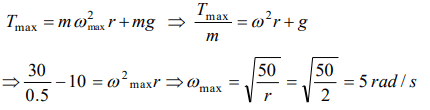## Motion in a Plane Questions and Answers Part-13

1. A bucket tied at the end of a 1.6 m long string is whirled in a vertical circle with constant speed. What should be the minimum speed so that the water from the bucket does not spill, when the bucket is at the highest position $\left(g=10m\diagup sec^{2}\right)$
a) 4 m/sec
b) 6.25 m/sec
c) 16 m/sec
d) None of the above

Explanation:2. A wheel is subjected to uniform angular acceleration about its axis. Initially its angular velocity is zero. In the first 2 sec, it rotates through an angle $\theta_{1}$ . In the next 2 sec, it rotates through an additional angle $\theta_{2}$ . The ratio of $\theta_{2}\diagup\theta_{1}$ is
a) 1
b) 2
c) 3
d) 5

Explanation:3. A 1 kg stone at the end of 1 m long string is whirled in a vertical circle at constant speed of 4 m/sec. The tension in the string is 6 N, when the stone is at $\left( g=10m\diagup sec^{2}\right)$
a) Top of the circle
b) Bottom of the circle
c) Half way down
d) None of the above

Explanation:4. A cane filled with water is revolved in a vertical circle of radius 4 meter and the water just doesnot fall down. The time period of revolution will be
a) 1 sec
b) 10 sec
c) 8 sec
d) 4 sec

Explanation:5. A 2 kg stone at the end of a string 1 m long is whirled in a vertical circle at a constant speed. The speed of the stone is 4 m/sec. The tension in the string will be 52 N, when the stone is
a) At the top of the circle
b) At the bottom of the circle
c) Halfway down
d) None of the above

Explanation:6. A body slides down a frictionless track which ends in a circular loop of diameter D , then the minimum height h of the body in term of D so that it may just complete the loop, is
a) $h=\frac{5D}{2}$
b) $h=\frac{5D}{4}$
c) $h=\frac{3D}{4}$
d) $h=\frac{D}{4}$

Explanation:7. A car is moving with speed 30 m/sec on a circular path of radius 500 m. Its speed is increasing at the rate of $2m\diagup sec^{2}$  . What is the acceleration of the car
a) $2m\diagup sec^{2}$
b) $2.7m\diagup sec^{2}$
c) $1.8m\diagup sec^{2}$
d) $9.8\diagup sec^{2}$

Explanation:8. The string of pendulum of length l is displaced through $90^{\circ}$ from the vertical and released. Then the minimum strength of the string in order to withstand the tension, as the pendulum passes through the mean position is
a) mg
b) 3mg
c) 5 mg
d) 6 mg

Explanation:9. A weightless thread can support tension upto 30 N. A stone of mass 0.5 kg is tied to it and is revolved in a circular path of radius 2 m in a vertical plane. If $g=10m\diagup sec^{2}$  , then the maximum angular velocity of the stone will be
b) $\sqrt{30}$ rad / s
c) $\sqrt{60}$ rad / sd) At an angle of $\cos^{-1}\left(1\diagup3\right)$   from the upward vertical